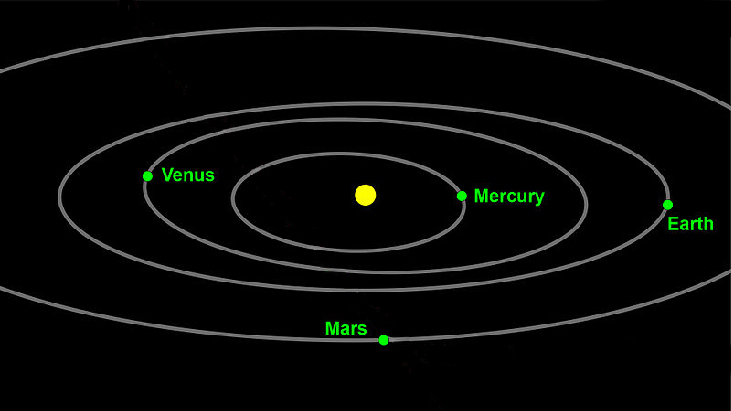# 8.4 Polar coordinates: graphs

 Page 1 / 16
In this section you will:
• Test polar equations for symmetry.
• Graph polar equations by plotting points.

The planets move through space in elliptical, periodic orbits about the sun, as shown in [link] . They are in constant motion, so fixing an exact position of any planet is valid only for a moment. In other words, we can fix only a planet’s instantaneous position. This is one application of polar coordinates    , represented as $\text{\hspace{0.17em}}\left(r,\theta \right).\text{\hspace{0.17em}}$ We interpret $\text{\hspace{0.17em}}r\text{\hspace{0.17em}}$ as the distance from the sun and $\text{\hspace{0.17em}}\theta \text{\hspace{0.17em}}$ as the planet’s angular bearing, or its direction from a fixed point on the sun. In this section, we will focus on the polar system and the graphs that are generated directly from polar coordinates.Planets follow elliptical paths as they orbit around the Sun. (credit: modification of work by NASA/JPL-Caltech)

## Testing polar equations for symmetry

Just as a rectangular equation such as $\text{\hspace{0.17em}}y={x}^{2}\text{\hspace{0.17em}}$ describes the relationship between $\text{\hspace{0.17em}}x\text{\hspace{0.17em}}$ and $\text{\hspace{0.17em}}y\text{\hspace{0.17em}}$ on a Cartesian grid, a polar equation describes a relationship between $\text{\hspace{0.17em}}r\text{\hspace{0.17em}}$ and $\text{\hspace{0.17em}}\theta \text{\hspace{0.17em}}$ on a polar grid. Recall that the coordinate pair $\text{\hspace{0.17em}}\left(r,\theta \right)\text{\hspace{0.17em}}$ indicates that we move counterclockwise from the polar axis (positive x -axis) by an angle of $\text{\hspace{0.17em}}\theta ,\text{\hspace{0.17em}}$ and extend a ray from the pole (origin) $\text{\hspace{0.17em}}r\text{\hspace{0.17em}}$ units in the direction of $\text{\hspace{0.17em}}\theta .\text{\hspace{0.17em}}$ All points that satisfy the polar equation are on the graph.

Symmetry is a property that helps us recognize and plot the graph of any equation. If an equation has a graph that is symmetric with respect to an axis, it means that if we folded the graph in half over that axis, the portion of the graph on one side would coincide with the portion on the other side. By performing three tests, we will see how to apply the properties of symmetry to polar equations. Further, we will use symmetry (in addition to plotting key points, zeros, and maximums of $\text{\hspace{0.17em}}r\right)\text{\hspace{0.17em}}$ to determine the graph of a polar equation.

In the first test, we consider symmetry with respect to the line $\text{\hspace{0.17em}}\theta =\frac{\pi }{2}\text{\hspace{0.17em}}$ ( y -axis). We replace $\text{\hspace{0.17em}}\left(r,\theta \right)\text{\hspace{0.17em}}$ with $\text{\hspace{0.17em}}\left(-r,-\theta \right)\text{\hspace{0.17em}}$ to determine if the new equation is equivalent to the original equation. For example, suppose we are given the equation $\text{\hspace{0.17em}}r=2\mathrm{sin}\text{\hspace{0.17em}}\theta ;$

This equation exhibits symmetry with respect to the line $\text{\hspace{0.17em}}\theta =\frac{\pi }{2}.$

In the second test, we consider symmetry with respect to the polar axis ( $\text{\hspace{0.17em}}x$ -axis). We replace $\text{\hspace{0.17em}}\left(r,\theta \right)\text{\hspace{0.17em}}$ with $\text{\hspace{0.17em}}\left(r,-\theta \right)\text{\hspace{0.17em}}$ or $\text{\hspace{0.17em}}\left(-r,\pi -\theta \right)\text{\hspace{0.17em}}$ to determine equivalency between the tested equation and the original. For example, suppose we are given the equation $\text{\hspace{0.17em}}r=1-2\mathrm{cos}\text{\hspace{0.17em}}\theta .$

The graph of this equation exhibits symmetry with respect to the polar axis.

In the third test, we consider symmetry with respect to the pole (origin). We replace $\text{\hspace{0.17em}}\left(r,\theta \right)\text{\hspace{0.17em}}$ with $\text{\hspace{0.17em}}\left(-r,\theta \right)\text{\hspace{0.17em}}$ to determine if the tested equation is equivalent to the original equation. For example, suppose we are given the equation $\text{\hspace{0.17em}}r=2\mathrm{sin}\left(3\theta \right).$

$\begin{array}{c}\text{\hspace{0.17em}}\text{\hspace{0.17em}}\text{\hspace{0.17em}}\text{\hspace{0.17em}}\text{\hspace{0.17em}}r=2\mathrm{sin}\left(3\theta \right)\\ -r=2\mathrm{sin}\left(3\theta \right)\end{array}$

The equation has failed the symmetry test , but that does not mean that it is not symmetric with respect to the pole. Passing one or more of the symmetry tests verifies that symmetry will be exhibited in a graph. However, failing the symmetry tests does not necessarily indicate that a graph will not be symmetric about the line $\text{\hspace{0.17em}}\theta =\frac{\pi }{2},\text{\hspace{0.17em}}$ the polar axis, or the pole. In these instances, we can confirm that symmetry exists by plotting reflecting points across the apparent axis of symmetry or the pole. Testing for symmetry is a technique that simplifies the graphing of polar equations, but its application is not perfect.

#### Questions & Answers

how fast can i understand functions without much difficulty
Joe Reply
what is set?
Kelvin Reply
a colony of bacteria is growing exponentially doubling in size every 100 minutes. how much minutes will it take for the colony of bacteria to triple in size
Divya Reply
I got 300 minutes. is it right?
Patience
no. should be about 150 minutes.
Jason
It should be 158.5 minutes.
Mr
ok, thanks
Patience
100•3=300 300=50•2^x 6=2^x x=log_2(6) =2.5849625 so, 300=50•2^2.5849625 and, so, the # of bacteria will double every (100•2.5849625) = 258.49625 minutes
Thomas
what is the importance knowing the graph of circular functions?
Arabella Reply
can get some help basic precalculus
ismail Reply
What do you need help with?
Andrew
how to convert general to standard form with not perfect trinomial
Camalia Reply
can get some help inverse function
ismail
Rectangle coordinate
Asma Reply
how to find for x
Jhon Reply
it depends on the equation
Robert
yeah, it does. why do we attempt to gain all of them one side or the other?
Melissa
whats a domain
mike Reply
The domain of a function is the set of all input on which the function is defined. For example all real numbers are the Domain of any Polynomial function.
Spiro
Spiro; thanks for putting it out there like that, 😁
Melissa
foci (–7,–17) and (–7,17), the absolute value of the differenceof the distances of any point from the foci is 24.
Churlene Reply
difference between calculus and pre calculus?
Asma Reply
give me an example of a problem so that I can practice answering
Jenefa Reply
x³+y³+z³=42
Robert
dont forget the cube in each variable ;)
Robert
of she solves that, well ... then she has a lot of computational force under her command ....
Walter
what is a function?
CJ Reply
I want to learn about the law of exponent
Quera Reply
explain this
Hinderson Reply

### Read also:

#### Get Jobilize Job Search Mobile App in your pocket Now!

Source:  OpenStax, Precalculus. OpenStax CNX. Jan 19, 2016 Download for free at https://legacy.cnx.org/content/col11667/1.6
Google Play and the Google Play logo are trademarks of Google Inc.

Notification Switch

Would you like to follow the 'Precalculus' conversation and receive update notifications?ByBy Yacoub JayoghliBy Yasser IbrahimBy OpenStaxBy OpenStaxBy OpenStaxBy OpenStaxByBy OpenStaxBy OpenStaxBy Rohini Ajay